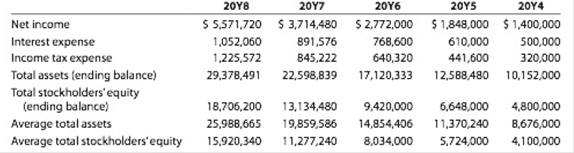# Solvency and profitability trend analysis Crosby Company has provided the following comparative information: You have been asked to evaluate the historical performance of the company over the last five years. Selected industry ratios have remained relatively steady at the following levels Tor the last five years: 20Y4-20Y8 Return on total assets 19% Return on stockholders’ equity 26% Times interest earned 3.4 Ratio of liabilities to stockholders’ equity 1.4 Instructions 1. Prepare four line graphs with the ratio on the vertical axis and the years on the horizontal axis for the following four ratios, rounding ratios and percentages to one decimal place: a. Return on total assets b. Return on stockholders’ equity c. Times interest earned d. Ratio of liabilities to stockholders’ equity Display both the company ratio and the industry benchmark on each graph. That is, each graph should have two lines. 2. Prepare an analysis of the graphs in (1).### Accounting

27th Edition
WARREN + 5 others
Publisher: Cengage Learning,
ISBN: 9781337272094### Accounting

27th Edition
WARREN + 5 others
Publisher: Cengage Learning,
ISBN: 9781337272094

#### Solutions

Chapter
Section
Chapter 17, Problem 17.5BPR
Textbook Problem

## Solvency and profitability trend analysisCrosby Company has provided the following comparative information:You have been asked to evaluate the historical performance of the company over the last five years.Selected industry ratios have remained relatively steady at the following levels Tor the last five years:   20Y4-20Y8 Return on total assets 19% Return on stockholders’ equity 26% Times interest earned 3.4 Ratio of liabilities to stockholders’ equity 1.4 Instructions1. Prepare four line graphs with the ratio on the vertical axis and the years on the horizontal axis for the following four ratios, rounding ratios and percentages to one decimal place: a. Return on total assets b. Return on stockholders’ equity c. Times interest earned d. Ratio of liabilities to stockholders’ equity Display both the company ratio and the industry benchmark on each graph. That is, each graph should have two lines.2. Prepare an analysis of the graphs in (1).

Expert Solution

1.

To determine

Financial Ratios: Financial ratios are the metrics used to evaluate the liquidity, capabilities, profitability, and overall performance of a company.

Given info: Items of financial statement

To determine: The following ratios for five years:

1. (a) Return on total assets
2. (b) Return on stockholders’ equity
3. (c) Times interest earned ratio
4. (d) Ratio of total liabilities to stockholders’ equity

### Explanation of Solution

Return on assets determines the particular company’s overall earning power. It is determined by dividing sum of net income and interest expense and average total assets.

Formula:

Rate of return&#

Expert Solution

2.

To determine

To prepare: Analysis of graphs

### Want to see the full answer?

Check out a sample textbook solution.See solution

### Want to see this answer and more?

Bartleby provides explanations to thousands of textbook problems written by our experts, many with advanced degrees!

See solution